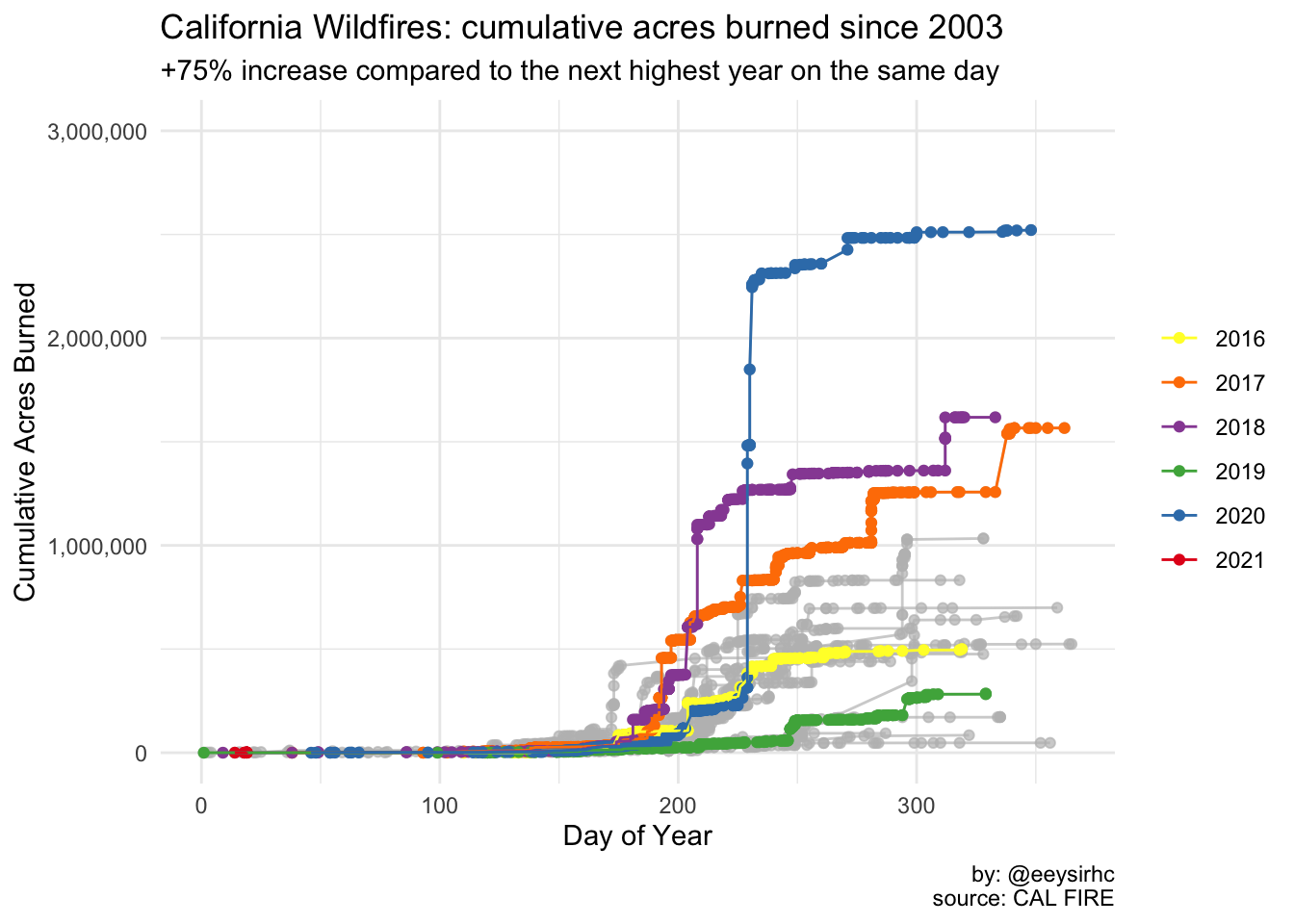Wildfires are raging across California (again).

What I noticed over the years of “doom watching” is how the news only report on tabulated data. They lacked any sort of visualization to underscore the impact of these fires.

Curiosity got the best of me so I searched around the CAL FIRE website and found a JSON endpoint for their incident data. The following code reveals how I created a graph in #rstats and used it as my first submission to the r/dataisbeautiful subreddit.

``````library(tidyverse)
library(lubridate)
library(scales)
library(jsonlite)
library(gghighlight)``````

``````wildfires_raw <- fromJSON("https://www.fire.ca.gov/umbraco/api/IncidentApi/List?inactive=true",
flatten = TRUE) %>% as_tibble()``````

## Parse data

``````wildfires <- wildfires_raw %>%
select(Name, County, Location, AcresBurned, IsActive,
StartedDateOnly, ExtinguishedDateOnly) %>%

# REMOVE INCORRECT DATA
filter(AcresBurned <= 100e6,
StartedDateOnly >= '2000-01-01') %>%

# CONVERT VARIABLES TO DATE FORMAT
mutate(StartedDateOnly = date(StartedDateOnly),
ExtinguishedDateOnly = case_when(ExtinguishedDateOnly == "" ~ as.character(StartedDateOnly),
TRUE ~ as.character(ExtinguishedDateOnly)),
ExtinguishedDateOnly = date(ExtinguishedDateOnly))

# COMPUTE CUMULATIVE TOTAL
wildfires_parsed <- wildfires %>%
mutate(year = year(StartedDateOnly),
day_index = yday(StartedDateOnly)) %>%
arrange(year, day_index) %>%
group_by(year) %>%
mutate(cumulative_acresburned = cumsum(AcresBurned)) %>%
ungroup()

# DIFFERENCE CALCULATION FOR GRAPH
calc <- wildfires_parsed %>% filter(day_index == 256,
year == 2020 | year == 2018) %>%
select(cumulative_acresburned)
burned_calc <- round(100 * (calc[2,] / calc[1,] - 1),2) %>% pull()``````

## Plot chart

``````wildfires_parsed %>%
ggplot(aes(day_index, cumulative_acresburned, color = factor(year))) +
geom_point() +
geom_line() +
gghighlight(year >= 2016) +
expand_limits(y = 0) +
scale_y_continuous(labels = comma_format(),
limits = c(0, 3e6)) +
scale_color_brewer(palette = 'Set1', direction = -1) +
labs(x = "Day of Year", y = "Cumulative Acres Burned", color = NULL,
title = "California Wildfires: cumulative acres burned since 2003",
subtitle = paste0("+", burned_calc, "% increase compared to the next highest year on the same day"),
caption = "by: @eeysirhc\nsource: CAL FIRE") +
theme_minimal() ``````## Top 20 California wildfires

I did not submit the one below but including it here to highlight how 2020 now accounts for 25% of California’s most devastating fires in the last two decades.

``````wildfires %>%
arrange(desc(AcresBurned)) %>%
top_n(20, AcresBurned) %>%
mutate(year = year(StartedDateOnly)) %>%
select(Name, year, AcresBurned) %>%
mutate(Name = reorder(Name, AcresBurned)) %>%
ggplot(aes(Name, AcresBurned, fill = factor(year))) +
geom_col() +
gghighlight(year >= 2016) +
coord_flip() +
labs(fill = NULL, x = NULL, y = "Total Acres Burned",
caption = "by: @eeysirhc\nsource: CAL FIRE") +
scale_x_discrete(labels = function(x) str_wrap(x, width = 45)) +
scale_y_continuous(labels = comma_format()) +
scale_fill_brewer(palette = 'Set1', direction = -1) +
theme_minimal()``````## Future work

• Use the {gganimate} package to rank the top 20 fires over time with a racing bar chart
• Build an interactive Shiny app which features a map, incident status, and other information
• Determine if wildfires are taking longer to extinguish than before by using survival analysis

For the last bullet point I contacted CAL FIRE over Twitter and email - waiting for some data corrections before I complete the survival regression.